# Catch up on the latest AI articles# A Smoothing Method For Robust Overfitting Suppression

3 main points
✔️ Introduce two smoothing methods to suppress Robust Overfitting
✔️ Use logit smoothing and weight smoothing as smoothing methods
✔️ Succeeded in improving standard accuracy and robust accuracy simultaneously

written by Tianlong ChenZhenyu ZhangSijia LiuShiyu ChangZhangyang Wang
(Submitted on 29 Sept 2020 (modified: 25 Feb 2021))
Published as ICLR 2021 Poster
Subjects: Machine Learning (cs.LG); Machine Learning (stat.ML)code：## Outline of Research

Adversarial Attacks are an attack technique against deep learning models. This attack is called Adversarial Example, in which the model is fed with small noises on top of normal data to make it misclassified. To prevent this attack, a learning method called Adversarial Training is considered to be effective, which is to train the Adversarial Example in addition to the normal training data in advance, so that the Adversarial Example can be classified correctly. The idea is to be able to classify the Adversarial Example correctly.

Although this adversarial training has achieved a certain level of success, it also has a drawback that it is prone to overfitting (called Robust Overfitting). To prevent Robust Overfitting, the authors introduced two smoothing methods and succeeded in improving both standard accuracy and robust accuracy simultaneously.

## related research

The adversarial attack is an attack to mislead the output of the model by adding some processing to the data input to the model.

A well-known countermeasure against adversarial attacks is called Adversarial Training. It improves the robustness of a model by training it not only with normal data but also with adversarial samples.

This article describes a related study to this paper, which shows that early termination is effective in preventing Robust Overfitting.

### logit smoothing

Logit smoothing refers to the smoothing of the output probability distribution in a classification model. In this paper, we perform logit smoothing using knowledge distillation with the same pre-trained model as a teacher. This is influenced by the paper that label smoothing, which is a known method of logit smoothing, is one of the special patterns of knowledge distillation.

### Weight smoothing

In this paper, we use a method called Stochastic Weight Averaging (SWA) to smooth the weights. This method is an approximation of a method called Fast Geometric Ensembling (FGE).

FGE is a method to improve the generalization performance of a model. 80% of the model has been trained, and the remaining 20% is trained using a unique learning rate scheduling. In the process of training the remaining 20%, the learning rate based on the original scheduling oscillates between large and small values several times. We store the weights of the model at the time when the learning rate reaches the smallest value, and use these weights to train the ensemble when all 20% of the models have been trained. This method was devised based on the discovery that local solutions to the loss function can be connected by a simple curve without changing the value of the loss too much.

SWA is a method that overcomes the disadvantage of FGE, which is that it requires multiple models for forecasting. Weights.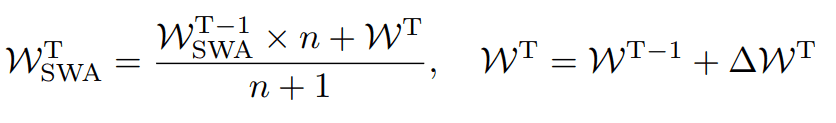In this way, we can approximate the performance of the FGE by updating it with the rule Since this method does not involve ensembling, it eliminates the need for multiple models and reduces the computational complexity of the prediction.

## proposed method

### Logit smoothing in Adversarial Training

The authors believe that Robust Overfitting is due in part to overfitting the Adversarial Example generated in the early stages of Adversarial Training. Therefore, the authors used logit smoothing to prevent overfitting to the initial Adversarial Example. Specifically, learning is performed by solving the following optimization problem.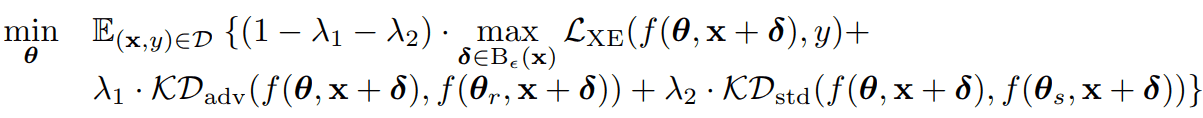We define the loss function as a weighted sum of three-loss functions: the loss function of ordinary adversarial training (first term), the loss function of knowledge distillation supervised by the adversarially trained model (second term), and the loss function of knowledge distillation supervised by the normally trained model (third term). The loss function is defined as the sum of the three-loss functions. By using this loss function, we can regularize ordinary Adversarial Training with the other two regularization terms (loss terms of knowledge distillation).

### Weight Smoothing in Adversarial Training

For weight smoothing, we use SWA, which can be directly used for adversarial training, andYou can implement this by simply incorporating this expression into your update rules.

## Experiments and Analysis

### Standard and Robust Accuracy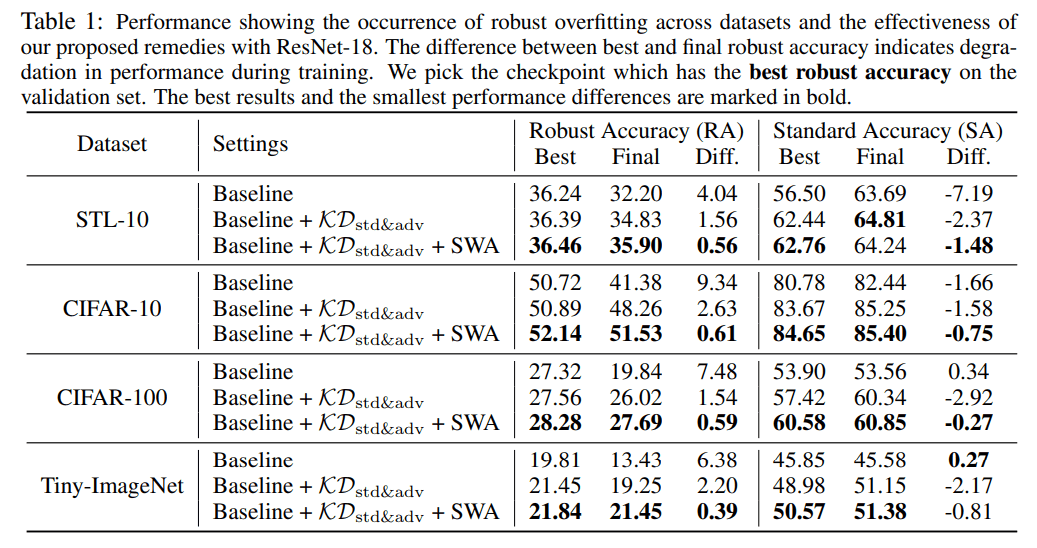For all the datasets, the model using the proposed method achieved the highest robust accuracy. Most of the standard accuracies also achieved the highest accuracy.

### Accuracy when varying attack methods

We prepared three types of attack methods, PGD, FGSM, and TRADES, and the accuracy for each of them is as follows.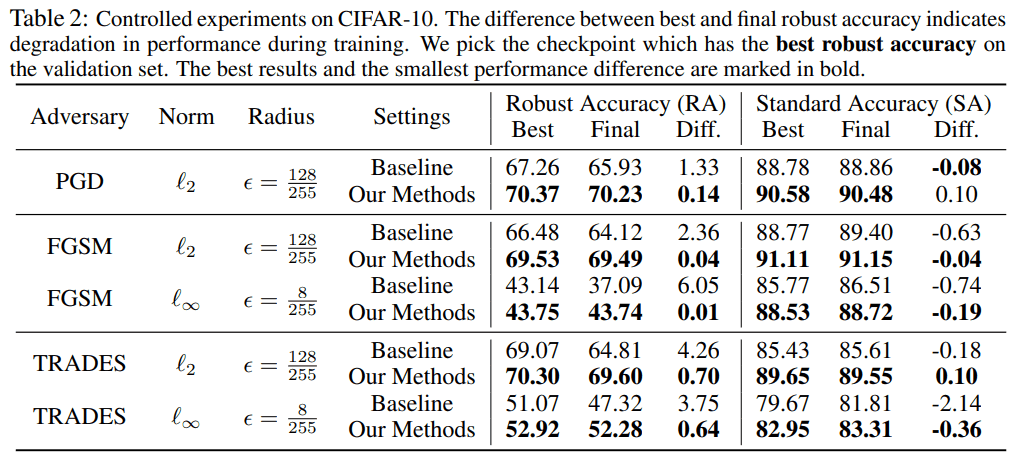For all the attack methods, the proposed method achieved higher values than the baseline in terms of robustness and standard accuracy.

### Accuracy on different architectures

For the three architectures VGG-16, WRN-34-4, and WRN-34-10, the accuracy when using the two datasets CIFAR-10 and CIFAR-100 are as follows.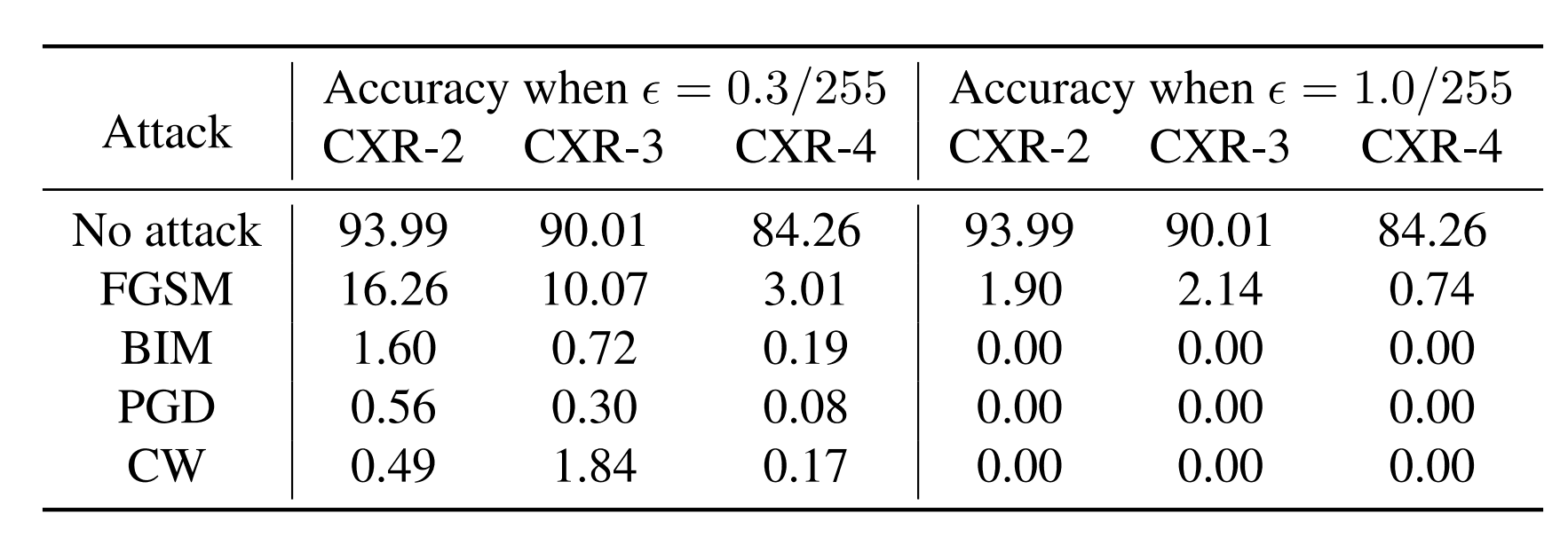All architectures achieve higher than baseline values along with robust and standard accuracy.

### Smoothing analysis

Smoothing of logit

The figure below shows how the data is captured by using logit smoothing using t-SNE.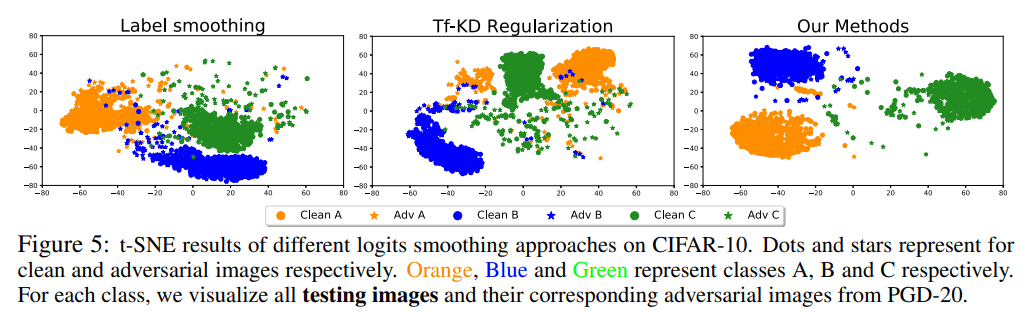Compared to other logit smoothing methods, such as label smoothing and Tf-KD (Teacher Free Knowledge Distillation), we can see that the separation is correct, including the Adversarial Example.

Smoothing of the loss function (smoothing of weights)

We checked whether the loss function could be smoothed by using the proposed method, and found that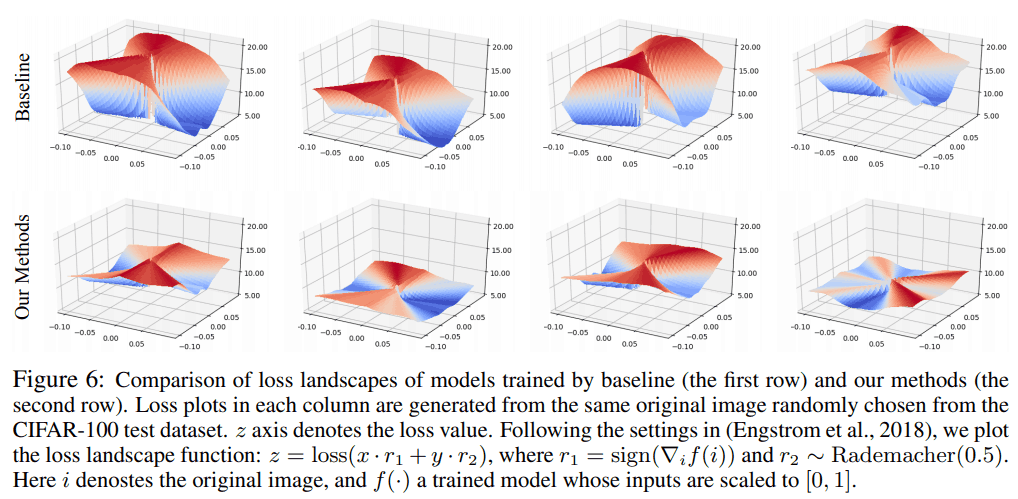It can be seen that the loss function is flattened when the proposed method is used.

## summary

In this paper, we proposed two smoothing methods, logit smoothing, and weight smoothing, as methods to prevent Robust Overfitting. By using these methods, we found that we can achieve higher robustness than before. However, the principle of Robust Overfitting is still unclear, so further research is needed.

If you have any suggestions for improvement of the content of the article,